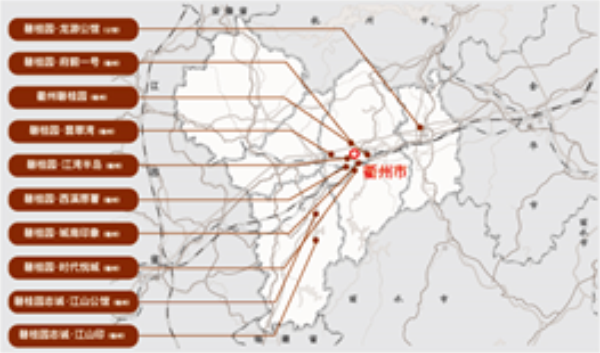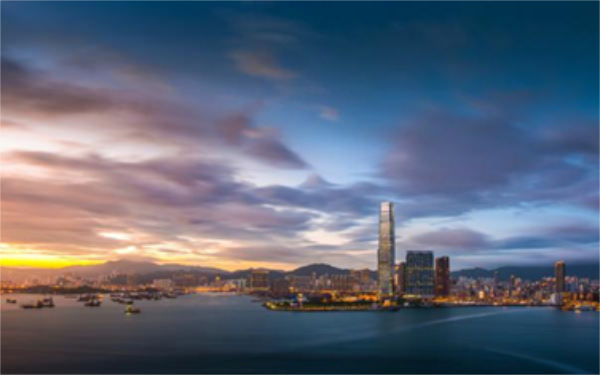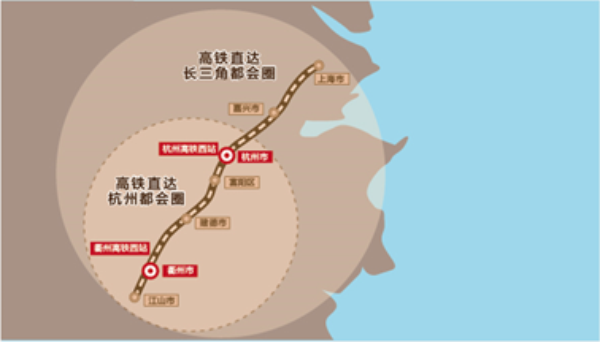## 网站地图## 碧桂园•江湾半岛

### 衢州

#### 地址

• 资阳
• 本溪
• 北流
• 万载
• 慈溪
• 长春
• 通化
• 西昌
• 丰城
• 阳春
• 鹤山
• 江油
• 辛集
• 辽源
• 衡水
• 黔西南州
• 黔东南州
• 石家庄
• 唐山
• 秦皇岛
• 邯郸
• 邢台
• 保定
• 张家口
• 承德
• 沧州
• 廊坊
• 黄骅
• 迁安
• 怀来
• 洛阳
• 驻马店
• 郑州
• 开封
• 平顶山
• 安阳
• 鹤壁
• 新乡
• 焦作
• 濮阳
• 许昌
• 漯河
• 三门峡
• 南阳
• 商丘
• 信阳
• 周口
• 武汉
• 黄石
• 十堰
• 宜昌
• 襄阳
• 荆州
• 荆门
• 鄂州
• 仙桃
• 潜江
• 黄冈
• 随州
• 咸宁
• 恩施
• 怀化
• 长沙
• 株洲
• 湘潭
• 湘西州
• 张家界
• 益阳
• 郴州
• 衡阳
• 岳阳
• 常德
• 娄底
• 徐州
• 南京
• 无锡
• 镇江
• 泰州
• 淮安
• 舟山
• 黔西南布依族苗族自治州
• 临沂
• 凉山
• 普洱
• 红河
• 锦州
• 葫芦岛
• 东方
• 咸阳
• 丽水
• 定安县
• 吕梁
• 来宾
• 河池
• 宜宾
• 重庆市
• 雅安
• 潮州
• 大理
• 巴中
• 桂林
• 贵港
• 百色
• 柳州
• 贺州
• 儋州
• 福清
• 威海
• 烟台
• 乐山
• 金华
• 永泰
• 潍坊
• 滨州
• 德州
• 临汾
• 日照
• 眉山
• 武威
• 乌鲁木齐
• 保山
• 临沧
• 防城港
• 达州
• 遂宁
• 绵阳
• 淮南
• 宝鸡
• 枣庄
• 桂平
• 景德镇
• 临夏
• 聊城
• 崇左
• 马鞍山
• 安顺
• 北海
• 菏泽
• 铜陵
• 黔南州
• 蚌埠
• 嘉兴
• 淮北
• 都匀
• 南宁
• 兴义
• 南平
• 松溪
• 吉安
• 大同
• 陆丰
• 厦门
• 铜仁
• 银川
• 昭通
• 包头
• 遵化
• 漳州
• 深圳
• 霸州
• 香港
• 合肥
• 抚州
• 琼海
• 定西
• 南昌
• 宿州
• 文昌
• 临高县
• 陵水
• 海口
• 博鳌
• 三亚
• 绥化
• 哈尔滨
• 杭州
• 绍兴
• 宁波
• 温州
• 衢州
• 湖州
• 台州
• 天津
• 沈阳
• 大连
• 通辽
• 兴安盟
• 呼伦贝尔
• 运城
• 晋中
• 太原
• 南通
• 宿迁
• 扬州
• 盐城
• 连云港
• 苏州
• 常州
• 安庆
• 黄山
• 巢湖
• 芜湖
• 池州
• 宣城
• 六安
• 古田
• 无为
• 桐城
• 亳州
• 南陵
• 庐江
• 阜阳
• 滁州
• 孝感
• 天门
• 邵阳
• 永州
• 平果
• 玉林
• 梧州
• 钦州
• 梅州
• 河源
• 云浮
• 东莞
• 汕头
• 中山
• 清远
• 惠州
• 韶关
• 肇庆
• 阳江
• 汕尾
• 茂名
• 江门
• 佛山
• 广州
• 雷州
• 英德
• 湛江
• 揭阳
• 珠海
• 信宜
• 济南
• 淄博
• 青岛
• 泰安
• 济宁
• 马来西亚雪兰莪州
• 马来西亚柔佛州
• 永春
• 南安
• 三明
• 宁德
• 武夷山
• 福鼎
• 莆田
• 建瓯
• 漳浦
• 福安
• 福州
• 泉州
• 龙岩
• 兰州
• 张掖
• 天水
• 白银
• 贵阳
• 仁怀
• 毕节
• 凯里
• 六盘水
• 遵义
• 广元
• 德阳
• 南充
• 泸州
• 攀枝花
• 广安
• 成都
• 宜春
• 萍乡
• 九江
• 赣州
• 上饶
• 曲靖
• 楚雄
• 昆明
• 白城
• 北京
• 澳洲莱德
• 西安
• 渭南
• 上海
• 西宁

7年10子 与衢州同发展碧桂园，世界500强，中国地产三强，中国新型城镇化进程的身体力行者，全国服务业主超400万。2013年首入衢州，已为约12000户家庭打造精品人居。

从东港到西区，碧桂园始终与衢州发展同行。2019年，政府重点发展区域内，碧桂园以7年深耕经验，打造第10子，带来人居新高度。2002年，以西区建设为标志，衢州从“南湖时代”迈入“衢江时代”。今天，西区已集行政办公、文化教育、商贸金融、会展商务、休闲居住等功能为一体，衢州繁华在此全面展现。西区发展也为衢州未来新一轮发展，奠定坚实基础。2018年10月，省发改委批复修建杭衢高铁;同月，衢州加入杭州都市圈;2019年2月，杭衢高铁开建。杭衢同城化，衢州新发展。恰逢机遇，“核心圈层”迎来发展蝶变。

“核心圈层”总用地面积约12平方公里，将定位为城市阳台打造，比肩杭州钱江新城、上海外滩。2017年，市政府提出启动城市核心圈层引爆点;2018年，“三城记”将其提到更高战略位置，“核心圈层”发展启动。百家塘片区作为“核心圈层”内珍罕一线江景居住板块，未来发展强劲。

市政府规划方案中，严家淤岛为“核心圈层”核芯，也是衢州发展的核芯眼。百家塘片区咫尺对望严家淤岛，严家淤岛既是百家塘的景观资源，更是未来发展共享者，一体共荣。

西区-“核心圈层”，碧桂园·江湾半岛立于百家塘，与严家淤岛仅咫尺约160米，无缝对接。同时，更以一线望江，三江口祗此两面环江低密住区，成就衢州人居高端封面。严家淤岛内规划约15万方信安湖活力岛，与碧桂园·江湾半岛一江两岸对望，预计于2019年动工，规划打造集生态旅游、文化体验、体育健身于一体的生态健康活力岛。

信安湖活力岛(规划)的建设、运营，将推动板块发展再迭变。碧桂园·江湾半岛业主将成板块发展主要影响者。

衢州实验学校新湖校区规模为60个教学班，以办学质量在衢州拥有不菲声誉。孩子入读好学校，拥抱璀璨未来。社区同时配建占地5000㎡的12班制幼儿园，畅享完善教育链。碧桂园·江湾半岛东侧打造196亩浙西健康城，以柯城区人民医院现址为核心，集合基本医疗、高端医疗及高端康养，全生命周期健康服务。同时，北面西区二期修建208亩(一期)四省边际中心医院(衢州市人民医院)。碧桂园·江��半岛近享双高端医疗服务，同时因江阻隔，不受医院区域复杂人流干扰，舒适宜居。